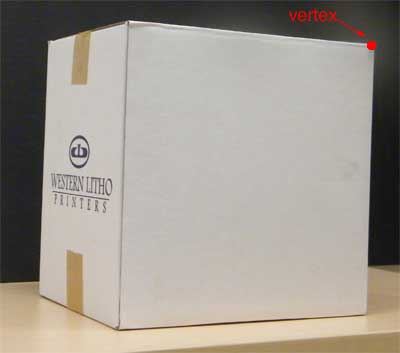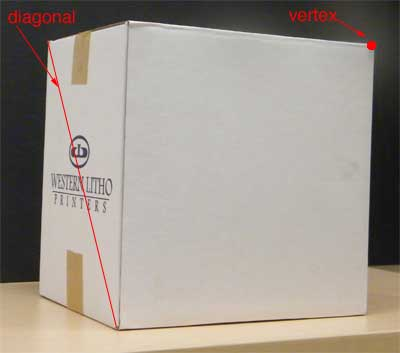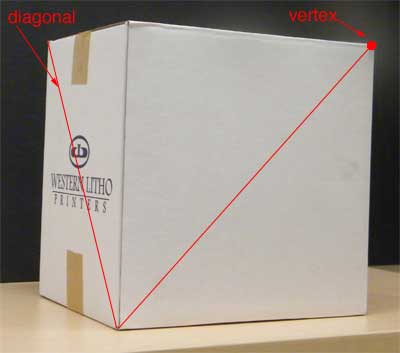SEARCH HOMEMath Central Quandaries & QueriesQuestion from tom, a student: a) Pass a plane containing a vertex of a rectangular parallelepiped and a diagonal of a face not containing that vertex to cut a pyramid from the parallelepiped. What fraction of the volume of the parallelepiped is the volume of the pyramid thus cut off? b)Pass a plane through a cube of edge 8 in so that the section formed will be a regular hexagon. Through each side of the hexagon pass two planes, one plane containing one of the two vertices of the cube which are farthest away from the plane of the hexagon, the other plane containing the diagonally opposite vertex of the cube. Find the volume of the solid bounded by these planes. c)three of the edges of a rectangular parallelepiped that meet in a point are also the lateral edges of a pyramid. What fraction of the parallelepiped is this pyramid...? you know the my only problem is how to illustrate this one...if only i could ...can you help me....all I need is the drawing and I can do the rest ..it's fine if you'll only answer any of these but I'll be thankful if you will do the three :)....Tom,

For problems involving a three dimensional object I find it helpful to have a physical model. A rectangular parallelepiped is a "box" and so I located a box in my office as a model for your first problem. The red dot identifies a vertex and the face with the printing does not contain the vertex.This face has two diagonals so there are two ways to cut the box. Here is one diagonal.Cutting the box by a plane containing this diagonal and the specified vertex cuts it along the red lines.This cuts the box into two pieces. Is either of the pieces a pyramid? What is its volume?

What happens if you cut along the other diagonal?

PennyMath Central is supported by the University of Regina and The Pacific Institute for the Mathematical Sciences.